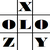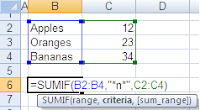anything in Excel...

It's Excel-o-lozy...

### Trick - "Sumif" - Advanced usage=Sumif (Range, Criteria, Sum range)
Range - data where criteria is to be applied (B2:B4)
Criteria* - conditions required ("*n*")
Sum range - data to be summed up ("C2:C4")
=Result = 57

Trick
*Criteria can be anything i.e. whole text or part of text.
In the example supra, I'v calculated Value of fruits based on condition that name of fruit shud contain "n".
I.e. any cobination of wild character can be used as criteria as follows: